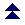Index

# Motion: Random..

These are the statements for random motion. There are horizontal and vertical parameters, and the statement RandomMove sets the bot in motion. If the bot is outside the random area, it will proceed in straight motion towards the random centre until it reaches the area. You must set the random motion parameters first, then issue the RandomMove statement.
 RandomCentre @c RandomCenter @c the central point of the random movement. If the bot is following or joining someone, only the altitude in @c will count, it will be used by the bot to calculate the altitude above the feet of the followed one, who will then act as centre of motion. If you wish the bot to stop following, issue the Stop statement before issuing the RandomMove statement. RandomHRadius %r maximum horizontal distance (m) the bot may move away from the random centre. Actually, it may move one step further. RandomHStep %s horizontal distance (m) the bot moves in one second before calculating a new random position. For humans, 0.75 to 1.5, for birds 1.5 to 2.5 or more. RandomHShift %a a fixed horizontal angle (degrees) to deviate from straight motion. Usually set to 0 (no fixed deviation) RandomHAngle %a maximum random horizontal angle (degrees) allowed to deviate from the fixed deviation angle. The larger the angle, the more chaotic the movement. RandomVHeight %a maximum random vertical deviation above the random centre. Make it 0 if you don't want the bot to move up and down. Used for flying avatars. For birds I use 4 to 10 metres. RandomVStep %a maximum random vertical step (m). For birds 1 or 2 meters. RandomWait %a Usually set to 0. On rare occasions, I change this to allow the bot to wait %a seconds between steps. RandomMove Starts the random movement. I usually precede this statement with the Stop statement to stop any follow/join movement. To stop the random movement, issue the Stop statement.

As from version 3.304, xelagots can sense terrain if terrain is available. Terrain sensing is OFF by default for random motion. The following statements apply:

 RandomTerSensing ON RandomTerSensing OFF Enables or disables terrain sensing when executing random motion. Default: OFF RandomTerMinAlt %a Sets the minimum altitude the bot must follow above the terrain (in metres), i.e. it may not go lower than this value relative to the terrain. The default is 0. If both maximum and minimum are 0, the bot will follow the terrain practically touching it. Notice that on slopes, the bot will be slightly above the terrain (as in the browser) if both values are 0 so as to keep the feet above the ground. For bird-bots, you may want to set this value to maybe 2 or 3 metres, so that the bird does not touch the terrain. RandomTerMaxAlt %a Sets the maximum altitude the bot must follow above the terrain (in metres). The default is 0. If the maximum altitude is lower than the minimum altitude, it is disregarded and the bot is not limited by a maximum altitude above the terrain (no maximum limit).Index